Previous in Forum: Is the Universe Really Expanding? Next in Forum: Noisy Furnace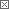Active Contributor

Join Date: Jan 2017
Posts: 14

#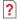Time-Time Component of FRW Metric

01/28/2017 3:43 AM

I understand that, in a given rest frame, standard clocks & rulers always span a constant metric interval. Adopting the MTW sign convention (-+++):

clocks from cycle to cycle span ds2 = -1

rulers from end to end span ds2 = +1

Particularly, when viewed from a valid embedding hyper-space, clocks always span the same hyper-spatial metric distance of one normalized unit. Even when the fabric of space-time warps, stretches, or shrinks, around them.

So, please ponder the usual FRW coordinate mesh (t,r) mapped into our ever-so-slightly closed space-time fabric. The *spatial* curvature requires:

ds2 = grr dr2 = dr2 / (1-r2) > dr2

because you can fit more standard rulers into one unit of differential *coordinate* distance dr, due to the curvature of the *spatial* slices of the space-time fabric.

But, by like logic, the *temporal* slices of space-time are also curved. For constant r, the time-like world-lines of rest-frame clocks move both "forward" in the direction of increasing *coordinate* time t, and also "outward" in the direction of increasing radius-of-curvature scale factor a(t). That requires:

ds2 = gtt dt2 = dt2 + da2 = (1 + (da/dt)2) dt2 > dt2

because you can fit more standard clock cycles into one unit of differential *coordinate* time dt, due to the rest-frame world-line moving "hyper-spatially outward" with the expansion of space-like slices of space-time, whose radius-of-curvature a(t) is inexorably increasing.

For all of those reasons, I tried modifying the FRW metric:

gtt = 1 → 1 + (da/dt)2

The equations resulting from the Einstein tensor equation, namely the (tt) & (rr) components thereof, remain tractable & elegant, and the resulting curve of a(t) vs. t is qualitatively similar to the standard result. However, when integrating the resulting equation:

f(a) da = dt

one finds that f(a) → 0 when a=0.2-0.3 for reasonable choices of curvature, matter, and radiation densities. f(a) becomes imaginary for small scale factors, and so I found it impossible to construct a solution which squeezes all the way down to a singular Big Bang at a=0.

Even if not particularly popular, I still hope that this suggested modification of the FRW metric's time-time component stimulates valuable discussion, one capable of explaining why it is not (or perhaps really actually is???) valid.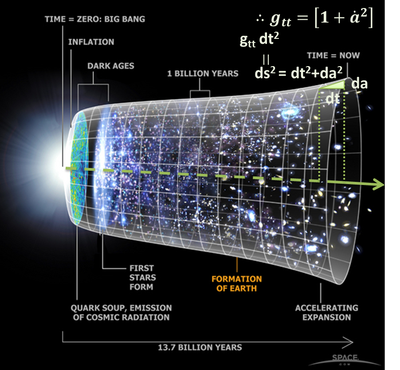Interested in this topic? By joining CR4 you can "subscribe" to
Associate

Join Date: May 2016
Location: Ohio, USA
Posts: 52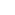#1

###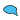Re: Time-Time component of FRW metric

01/28/2017 7:57 AM

Close to "Off topic response "

The speed of light could have been much faster in the early moments of cosmological time. Exponentially growing slower with age.

"Temporal Slices" are like growth spurts. Knowing the intake of nutrition "constant r" will only

gtt = d/dt (x/γ) = x'/γ - x γ'/γ2 1 to -1 → 1 + (da/dt)2

As you have stated in previous posts. perceptions of not even moving.

I don't intend to diminish or make fun of your post ! it was a good read.

and in sighted good conversation...

:)

__________________
If you cant say anything nice , dont say anything at all.
Active Contributor

Join Date: Jan 2017
Posts: 14#2

###Re: Time-Time component of FRW metric

02/04/2017 5:10 AM

Well, yours is the best reply yet !I tried more tinkering with my Mathematica models, turns out that modifying the time-time component of the metric:

gtt = -1 → -(1 - (da/dt)2)

actually can generate results similar to the canonical model and consistent with SNIa observations.

Please remember from SR that moving observers experience a time dilation by the factor of 1/γ such that their standard clocks appear "stretched" by that amount on an (x,t) diagram. So if we imagine that "hyper-spatial" motion in the "hyper-spatial radius-of-curvature direction" effects the same "stretch", then we have:

gtt = -1 → -γ-2

which is the above. If you choose ΩM≈0.3 then, b/c of the modified metric and resultingly modified Friedmann equations, you need to choose ΩΛ≥6 to fit SNIa data and "inflate" the solution up to reasonable sizes and universe life-times comparable to the known Hubble values.

Again b/c of the modified FRW equations, ΩΛ≥6 might not mean that the universe is so super-critical that the CMB would have to be wildly distorted. But even if that above hypothesis were correct, the discussion would require a researcher with the right connections to the right channels to pursue the same.

gtt = -γ-2 generates an interesting family of solutions which generally resemble canonical empty vacuum deSitter solutions, b/c the modified t-t component won't allow the "hyper-spatial expansion velocity" da/dt > c. So maybe they describe the fabric we actually live in ??